Test: Graph Theory- 2

# Test: Graph Theory- 2 - Electrical Engineering (EE)

Test Description

## 12 Questions MCQ Test GATE Electrical Engineering (EE) 2024 Mock Test Series - Test: Graph Theory- 2

Test: Graph Theory- 2 for Electrical Engineering (EE) 2023 is part of GATE Electrical Engineering (EE) 2024 Mock Test Series preparation. The Test: Graph Theory- 2 questions and answers have been prepared according to the Electrical Engineering (EE) exam syllabus.The Test: Graph Theory- 2 MCQs are made for Electrical Engineering (EE) 2023 Exam. Find important definitions, questions, notes, meanings, examples, exercises, MCQs and online tests for Test: Graph Theory- 2 below.
Solutions of Test: Graph Theory- 2 questions in English are available as part of our GATE Electrical Engineering (EE) 2024 Mock Test Series for Electrical Engineering (EE) & Test: Graph Theory- 2 solutions in Hindi for GATE Electrical Engineering (EE) 2024 Mock Test Series course. Download more important topics, notes, lectures and mock test series for Electrical Engineering (EE) Exam by signing up for free. Attempt Test: Graph Theory- 2 | 12 questions in 30 minutes | Mock test for Electrical Engineering (EE) preparation | Free important questions MCQ to study GATE Electrical Engineering (EE) 2024 Mock Test Series for Electrical Engineering (EE) Exam | Download free PDF with solutions
 1 Crore+ students have signed up on EduRev. Have you?
Test: Graph Theory- 2 - Question 1

### Let G be an arbitrary graph with n nodes and k components. If a vertex is removed from G, the number of components in the resultant graph must necessarily lie between.

Detailed Solution for Test: Graph Theory- 2 - Question 1

Maximum components will result after removal of a node if graph G is a star graph as shown below.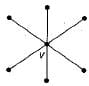or a null graph of n vertices as shown below: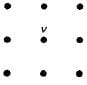In either case, if node vis removed, the number of components will be n - 1, where, n is the total number of nodes in the star graph.
∴ n - 1 is the maximum number of components possible. Minimum components will result if the node being removed is a lone vertex in which case, the number of components will be k - 1.
∴ The number of components must necessarily lie between k - 1 and n - 1.

Test: Graph Theory- 2 - Question 2

### How many perfect matchings are there in a complete graph of 6 vertices?

Detailed Solution for Test: Graph Theory- 2 - Question 2

The number of perfect matchings in a complete graph of n vertices, where n is even, reduces to the problem of finding unordered partitions of the vertex set of the type p(2n; 2, 2, 2, ... n times)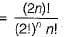For n = 3, 2n = 6, i.e. complete graph K6, we have
Number of perfect matchings =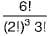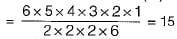Test: Graph Theory- 2 - Question 3

### A Graph G = (V, E) satisfies |E| ≤ 3 |V| - 6. The min-degree of G is defined as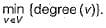Therefore, min-degree of G cannot be

Detailed Solution for Test: Graph Theory- 2 - Question 3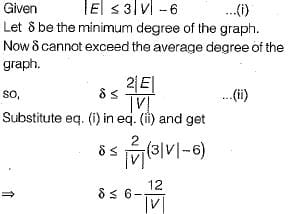Clearly the minimum degree has to be less than 6 always and hence cannot be equal to 6.

Test: Graph Theory- 2 - Question 4

What is the number of vertices in an undirected connected graph with 27 edges, 6 vertices of degree 2, 3 vertices of degree 4 and remaining of degree 3?

Detailed Solution for Test: Graph Theory- 2 - Question 4

Sum of degree of all vertices = 2e (using Handshaking lemma)
So, 6 x 2 + 3 x 4 + x x 3 = 2 x 27
12 + 12 + 3x = 54
3x = 54 - 24
x = 30/3
x = 10
So, total number of vertices
⇒ x + 6 + 3 = 10 + 6 + 3 = 19

Test: Graph Theory- 2 - Question 5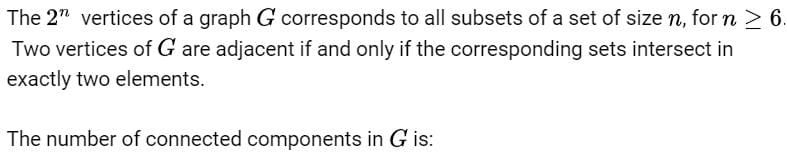Detailed Solution for Test: Graph Theory- 2 - Question 5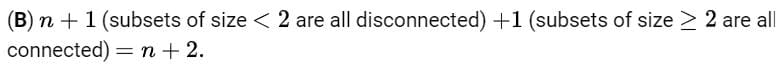Test: Graph Theory- 2 - Question 6

Which of the following graphs has an Eulerian circuit?

Detailed Solution for Test: Graph Theory- 2 - Question 6

Whenever in a graph all vertices have even degrees, it will surely have an Euler circuit.
(a) Since in a k-regular graph, every vertex has exactly k degrees and if k is even, every vertex in the graph has even degrees, kregular graph need not be connected, hence k-regular may not contain Euler circuit.
(b) Complete graph on 90 vertices not contains an Euler circuit, because every vertex degree is odd (89).
(c) C25 has 24 edges and each vertex has exactly 2 degrees. So every vertex in the complement of C25 will have 24 - 2 = 22 degrees which is an even number.
Since every vertex in the complement of C25 has even degrees. Also, the complements of all Cn with n ≥ 5 is connected. So, it is an Euler graph.

Test: Graph Theory- 2 - Question 7

G is a simple, connected, undirected graph. Some vertices of G are of odd degree. Add a node v to G and make it adjacent to each odd degree vertex of G. The resultant graph is sure to be

Detailed Solution for Test: Graph Theory- 2 - Question 7

In an undirected simple, connected graph number of vertices must be even of odd degree (using Handshaking lemma).
Adding a vertex v, adjacent to all odd degree vertices in graph, so degree of all odd degree vertices now become even and degree of vertex v is also even (since number of odd degree vertex are even).
Now all vertices in the graph are of even degree and graph is connected, so it must be Eular graph.

Test: Graph Theory- 2 - Question 8

The degree sequence of a simple graph is the sequence of the degrees of the nodes in the graph in decreasing order. Which of the following sequences can not be the degree sequence of any graph?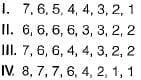Detailed Solution for Test: Graph Theory- 2 - Question 8

Havell-Hakimi algorithm can be used to check whether a given degree sequence is a graph or not.
The algorithm is
1. Remove top node of the sequence.
2. Subtract “1 ” from as many nodes in remaining sequence as the degree of top node that was removed.
3. Rearrange this sequence in non increasing order.
4. Check if resulting sequence is a graph.
5. Proceed again to step 1.
If the given sequence is not a graph we will see a violation in step 4, such as presence of negative degrees in the sequence. Otherwise the algorithm will bottom out with a degree sequence consisting of only even number of 1’s and any number of 0’s.
Now applying the algorithm to the degree sequences I, II, III and IV, one by one:
1.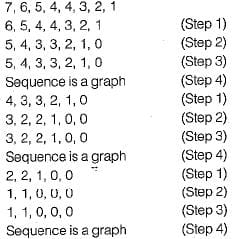Now, the algorithm ends, since the sequence has only 0’s and even number of 1’s.
The final sequence corresponds to following valid graph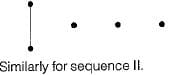2.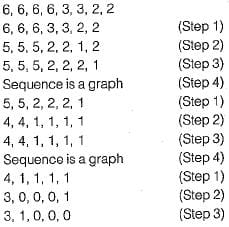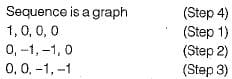The sequence is not a graph (Step 4), since negative degrees not possible in a valid graph. So, algorithm ends.
II is cannot be the degree sequence of any.graph. Similarly we can show that III is degree sequence of some graph and IV is not a degree sequence of any graph.

Test: Graph Theory- 2 - Question 9

Which of the following graphs is isomorphic to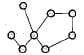Detailed Solution for Test: Graph Theory- 2 - Question 9

Check invariants are one by one.
Step 1: All 4 choice have same number of vertices and edges as given graph.
Step 2: So we find degree sequence of given graph which is (1,1, 2, 2, 2, 2, 2, 4).
Degree sequence of graph in option (a) is (1, 1, 1, 2, 2, 2, 3, 4).
Degree sequence of graph in option (b) is (1, 1, 2, 2, 2, 2, 2, 4)
Degree sequence of graph in option (c) is (1, 1,2, 2, 2, 2, 3, 3)
Degree sequence of graph in option (d) is ( 1 , 1 , 2, 2, 2, 2, 2 , 4 ) .
So only options (a) and (c) are not isomorphic to given graph, since degree sequence of these graphs is not same as given graph.
Step 3: Now to decide between options (b) and (d), which one is isomorphic to given graph, we check the number of cycles.
In given Graph there is one cycle of length 5 but in Graph (d), there is no cycle of length 5.
Graph (b) has one cycle of length 5.
So only Graph (b) can be isomorphic to given Graph.

Test: Graph Theory- 2 - Question 10

Which of the following statements is/are TRUE for undirected graph?
P: Number of odd degree vertices is even.
Q: Sum of degrees of all vertices is even.

Detailed Solution for Test: Graph Theory- 2 - Question 10

Q is Hand-shaking theorem and hence true.
P is a corollory to the Hand-shaking theorem and hence also true.

Test: Graph Theory- 2 - Question 11

An ordered n-tuple (d1, d2, .... dn) with d1 ≥ d≥ ... ≥ dn is called graphic if there exists a simple undirected graph with n vertices having degrees d1, d2, ..., dn respectively. Which of the following 6-tuples is NOT graphic?

Detailed Solution for Test: Graph Theory- 2 - Question 11

Use the Havell-hakimi algorithm.
The sequence of steps in the algorithm for each graph is shown below: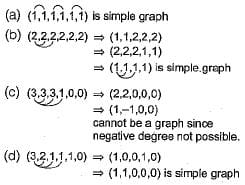Test: Graph Theory- 2 - Question 12

If G is a forest with n-vertices and k connected components, how many edges does G have?

Detailed Solution for Test: Graph Theory- 2 - Question 12

The number of edges in spanning forest of a graph G with n vertices and k components = Rank (G) = n - k .

## GATE Electrical Engineering (EE) 2024 Mock Test Series

23 docs|285 tests
Information about Test: Graph Theory- 2 Page
In this test you can find the Exam questions for Test: Graph Theory- 2 solved & explained in the simplest way possible. Besides giving Questions and answers for Test: Graph Theory- 2, EduRev gives you an ample number of Online tests for practice

## GATE Electrical Engineering (EE) 2024 Mock Test Series

23 docs|285 tests